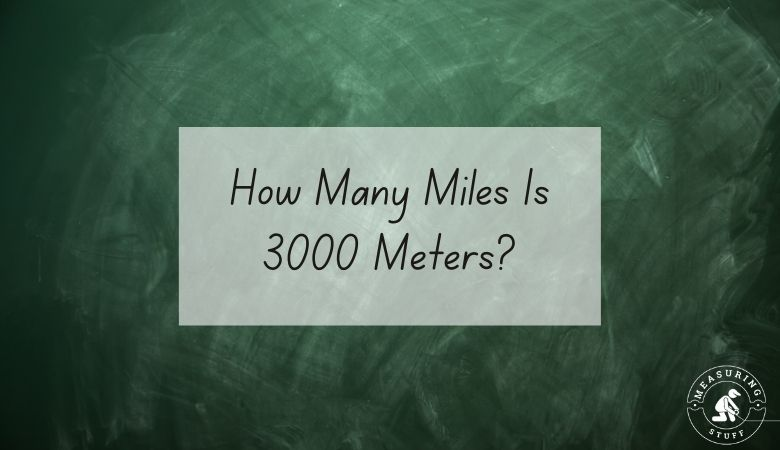# How Many Miles Is 3000 Meters?

There are 1.86451 miles in 3000 meters.

In order to find out how many miles are in a certain number of meters, you would need to divide the number of meters by 1,609.

For example, if you want to know how many miles are in 3000 meters, divide 3000 by 1,609.

The answer, which is 1.86451, tells you that there are that many miles in 3000 meters.

If you want to reverse the question and figure out how many meters can be made from a certain number of miles, you would multiply the number of miles by 1,609.

For example, if you have 3 miles, you can multiply it by 1,609 to get 4827.

This means that there are 4827 meters in 3 miles.

If you don’t feel like doing the math, use the meters into miles conversion calculator below.

Also, check out all of our online conversion calculators here.

You can also use the following table to convert meters into miles.

## Meters To Miles Conversion Table

You can also convert 3000 meters into other units of measurements.

• 3000 meters = 250 millimeters
• 3000 meters = 300000 centimeters
• 3000 meters = 118110 inches

Other articles on our site using meters and miles include:

## Meters And Miles Measurements

Meters are part of the metric system of measurement, which is common around the world thanks to its ease of use.

Although the metric system isn’t standard in the United States, it’s still commonly used for scientific measurements.

Miles are part of the imperial system of measurement, which is standard in the United States.

## Related articles:

Check out a complete list of related articles in this category here.